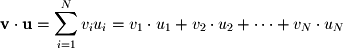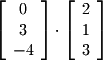A dot product, also known as the scalar product or inner product, is one generalization of scalar multiplication to higher dimensions. Like vector/vector addition and subtraction, it is accomplished by multiplying corresponding entries in two vectors, but unlike addition and subtraction, the output is a scalar quantity rather than a vector because after multiplying entries, the sum of the products is computed:From this definition it follows that dot products can be computed only for two vectors which have the same number of rows.
Excercise 2-5.
Find the following dot product: